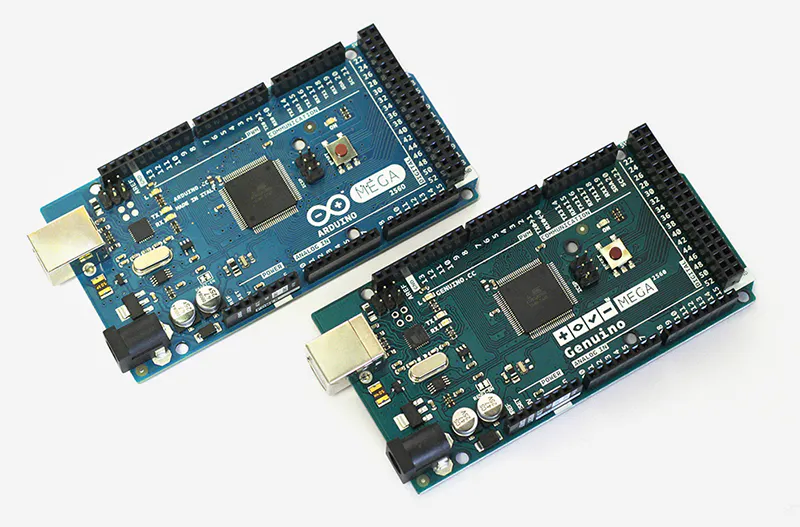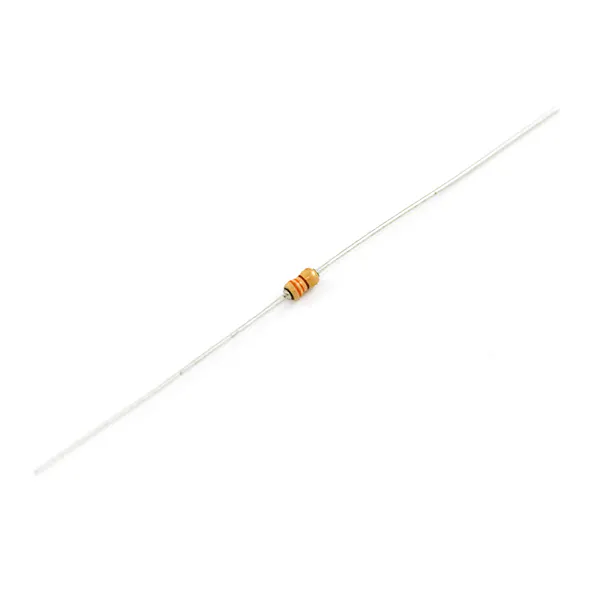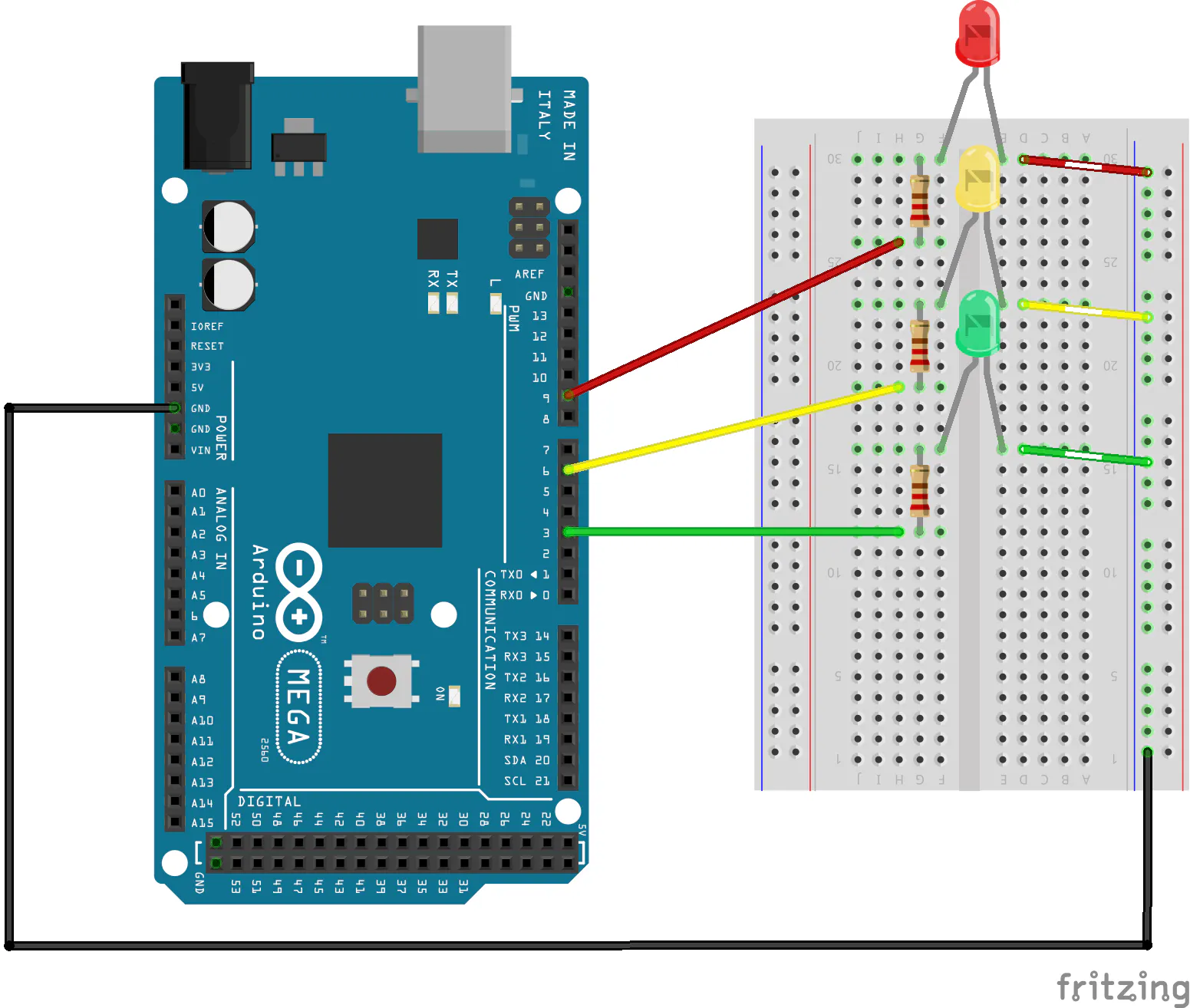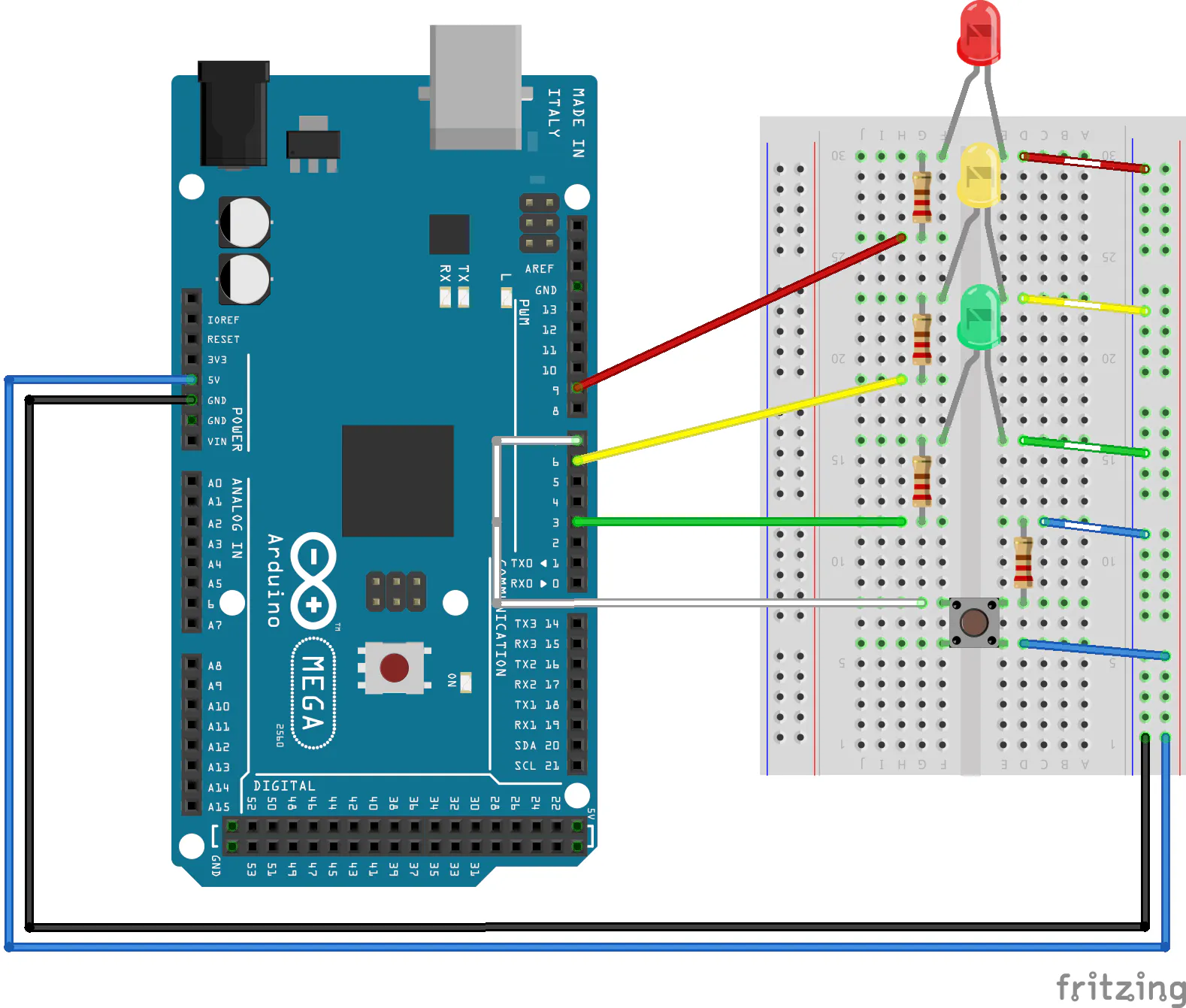# Dimming Lights with PWM using Push Button

This project will show you how to fade lights, add a button to it.

BeginnerFull instructions provided1 hour9,324## Things used in this project

### Hardware componentsArduino Mega 2560 & Genuino Mega 2560
×1×1Jumper wires (generic) The amount you use may differ, it depends on the method that is used.
×11LED (generic) Any colors would do, but I recommend 3 different colors.
×3SparkFun Pushbutton switch 12mm
×1Resistor 330 ohm
×4

### Software apps and online servicesArduino IDE

## Schematics### Fade Lights with Push Button## Code

Arduino
```int ledRed = 9;
int ledYellow = 6;
int ledGreen = 3;

void setup() {
pinMode(ledRed, OUTPUT);
pinMode(ledYellow, OUTPUT);
pinMode(ledGreen, OUTPUT);

}

void loop() {
//ledRed
for (int i = 0; i <= 255; i++) {
analogWrite(ledRed, i);
}
for (int i = 255; i >= 0; i--) {
analogWrite(ledRed, i);
}

//ledYellow
for (int i = 0; i <= 255; i++) {
analogWrite(ledYellow, i);
}
for (int i = 255; i >= 0; i--) {
analogWrite(ledYellow, i);
}

//ledGreen
for (int i = 0; i <= 255; i++) {
analogWrite(ledGreen, i);
}
for (int i = 255; i >= 0; i--) {
analogWrite(ledGreen, i);
}

//ledYellow
for (int i = 0; i <= 255; i++) {
analogWrite(ledYellow, i);
}
for (int i = 255; i >= 0; i--) {
analogWrite(ledYellow, i);
}

}
```

### Fade Lights with Push Button

Arduino
```int ledRed = 9;
int ledYellow = 6;
int ledGreen = 3;
int button = 7;

void setup() {
pinMode(ledRed, OUTPUT);
pinMode(ledYellow, OUTPUT);
pinMode(ledGreen, OUTPUT);
pinMode(button, INPUT);
digitalWrite(ledRed, LOW);
digitalWrite(ledYellow, LOW);
digitalWrite(ledGreen, HIGH);
}

void loop() {

delay(15);
}
} else {
digitalWrite(ledRed, LOW);
digitalWrite(ledYellow, LOW);
digitalWrite(ledGreen, HIGH);
}
}

for (int i = 255; i >= 0; i--) {
analogWrite(ledGreen, i);
}
//ledYellow
for (int i = 0; i <= 255; i++) {
analogWrite(ledYellow, i);
}
for (int i = 255; i >= 0; i--) {
analogWrite(ledYellow, i);
}

//ledRed
for (int i = 0; i <= 255; i++) {
analogWrite(ledRed, i);
}
for (int i = 255; i >= 0; i--) {
analogWrite(ledRed, i);
}

//ledYellow
for (int i = 0; i <= 255; i++) {
analogWrite(ledYellow, i);
}
for (int i = 255; i >= 0; i--) {
analogWrite(ledYellow, i);
}

//ledGreen
for (int i = 0; i <= 255; i++) {
analogWrite(ledGreen, i);
}

}
```

### Fade Lights with Push Button (improvement to delay)

Arduino
```int ledRed = 9;
int ledYellow = 6;
int ledGreen = 3;
int button = 7;

void setup() {
pinMode(ledRed, OUTPUT);
pinMode(ledYellow, OUTPUT);
pinMode(ledGreen, OUTPUT);
pinMode(button, INPUT);
digitalWrite(ledRed, LOW);
digitalWrite(ledYellow, LOW);
digitalWrite(ledGreen, HIGH);

}

void loop() {
delay(15);
}
} else {
digitalWrite(ledRed, LOW);
digitalWrite(ledYellow, LOW);
digitalWrite(ledGreen, HIGH);
}
}

for( int i=255; i>=0; i--) {
analogWrite(ledGreen, i);
for( int y = 0; y < 1000; y++){
// if button is released
return;
}
}
}

//ledYellow
for( int i=0; i<=255; i++) {
analogWrite(ledYellow, i);
for( int y = 0; y < 400; y++){
// if button is released
return;
}
}
}

for( int i=255; i>=0; i--) {
analogWrite(ledYellow, i);
for( int y = 0; y < 400; y++){
// if button is released
return;
}
}
}

//ledRed
for( int i=0; i<=255; i++) {
analogWrite(ledRed, i);
for( int y = 0; y < 1000; y++){
// if button is released
return;
}
}
}
for( int i=255; i>=0; i--) {
analogWrite(ledRed, i);
for( int y = 0; y < 1000; y++){
// if button is released
return;
}
}
}

//ledYellow
for( int i=0; i<=255; i++) {
analogWrite(ledYellow, i);
for( int y = 0; y < 400; y++){
// if button is released
return;
}
}
}

for( int i=255; i>=0; i--) {
analogWrite(ledYellow, i);
for( int y = 0; y < 400; y++){
// if button is released
return;
}
}
}

//ledGreen
for( int i=0; i<=255; i++) {
analogWrite(ledGreen, i);
for( int y = 0; y < 1000; y++){
// if button is released
return;
}
}
}
}
```

## Credits

### Phuong Vo

4 projects • 8 followers
Hi